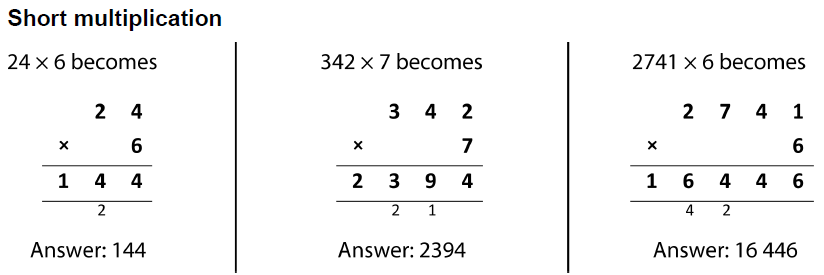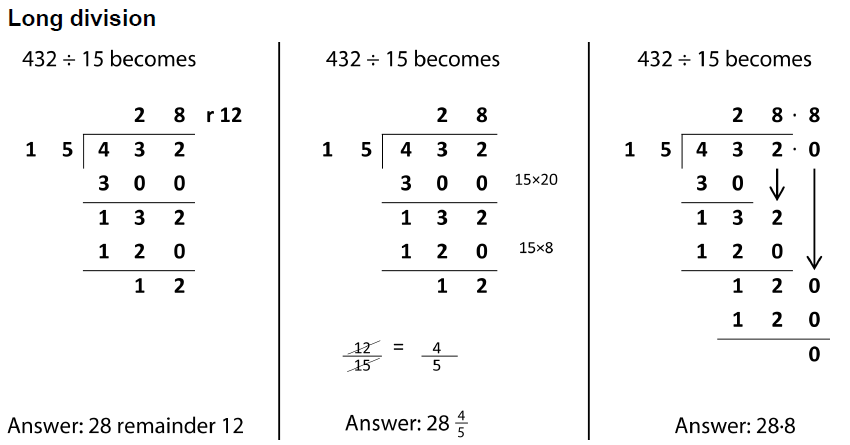Formal Written Methods

Examples of formal written methods for addition, subtraction, multiplication and division.

The following examples show formal written methods for all four operations to illustrate the range of methods that could be taught. It is not intended to be an exhaustive list, nor is it intended to show progression in formal written methods. For example, the exact position of intermediate calculations (superscript and subscript digits) will vary depending on the method and format used.Try Some!Try Some!Try Some!Try Some!Try Some!

Try Mixed

Examples above reproduced under the terms of the UK Open Government Licence. Reference: DFE-00180-2013

Extracts from the UK Year 5 and Year 6 programme of study:

Pupils should be taught to:

• add and subtract whole numbers with more than 4 digits, including using formal written methods (columnar addition and subtraction); more…
• multiply multi-digit numbers up to 4 digits by a two-digit whole number using the formal written method of long multiplication; more…
• divide numbers up to 4 digits by a two-digit number using the formal written method of short division where appropriate, interpreting remainders according to the context. more…
• divide numbers up to 4 digits by a two-digit whole number using the formal written method of long division, and interpret remainders as whole number remainders, fractions, or by rounding, as appropriate for the context; more…

There are also more resources than you can shake a stick at on the Arithmetic topic page.

Do you have any comments? It is always useful to receive feedback and helps make this free resource even more useful for those learning Mathematics anywhere in the world. Click here to enter your comments.For All: